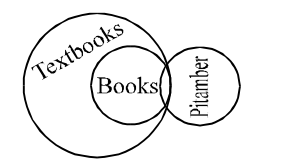## Syllogism

#### Syllogism

Direction: In each of the question below are three statements following by three conclusions numbered I, II and III. You have to take the three given statements to be true even if they seem to be at variance with commonly known facts and then decide which of the given conclusion logically follows from the two statements disregarding commonly known fact.

1. Statement:
All buildings are mirrors. Some mirrors are pens. No pen is paper.
Conclusions:
I. Some papers are buildings.
II. Some pens are buildings.
III. Some papers are mirrors.

1. Some mirrors are pens + No pen is paper = 1 + E = O* = Some mirrors are not papers Hence III does not follow. All buildings are mirrors + Some mirrors are pens = A + I = No conclusion Hence II and consequently I do not follow.

##### Correct Option: A

Some mirrors are pens + No pen is paper = 1 + E = O* = Some mirrors are not papers Hence III does not follow. All buildings are mirrors + Some mirrors are pens = A + I = No conclusion Hence II and consequently I do not follow.

1. Statement:
Some books are trees. All tress are roads. All roads are wheels.
Conclusions:
I. Some wheels are books.
III. Some wheels are trees.

1. Some books are trees + All tress are roads = I + A = I = Some books are roads (I) Hence II follows. Some books are trees + All roads are wheels = I + A = I = Some books are wheels → conversion → Some wheels are books (I) Hence I follows. All trees are roads + All roads are wheels = A + A = A = All trees are wheels → conversion → Some wheels are trees (I) Hence III follows.

##### Correct Option: D

Some books are trees + All tress are roads = I + A = I = Some books are roads (I) Hence II follows. Some books are trees + All roads are wheels = I + A = I = Some books are wheels → conversion → Some wheels are books (I) Hence I follows. All trees are roads + All roads are wheels = A + A = A = All trees are wheels → conversion → Some wheels are trees (I) Hence III follows.

1. Statement:
All stones are rivers. All rivers are cars. Some cars are trains.
Conclusions:
I. Some trains are stones.
II. Some cars are stones.
III. Some trains are rivers.

1. All stones are rivers + All rivers are cars = A + A = A = All stones are cars → conversion → Some cars are stones (I) Hence II follows. All rivers are cars + Some cars are trains = I + A = No conclusion Hence II and consequently I do not follow.

##### Correct Option: C

All stones are rivers + All rivers are cars = A + A = A = All stones are cars → conversion → Some cars are stones (I) Hence II follows. All rivers are cars + Some cars are trains = I + A = No conclusion Hence II and consequently I do not follow.

1. Statement:
Some rivers are hills. No hill is taxi. All taxis are buses.
Conclusions:
I. Some buses are rivers.
II. Some taxis are rivers.
III. No bus is river.

1. Some rivers are hills + No hill is taxi = I + E = O = Some rivers are not taxis Hence II does not follow. Again, Since O-type statements can't be combined, neither I nor III follows. But the two form a complementary E- I pair, Hence either I or III follows.

##### Correct Option: E

Some rivers are hills + No hill is taxi = I + E = O = Some rivers are not taxis Hence II does not follow. Again, Since O-type statements can't be combined, neither I nor III follows. But the two form a complementary E- I pair, Hence either I or III follows.

1. In the following question a statement is followed by four inferences. Select the one which is most appropriate.
All the books, written by Prabhakar, are textbooks.

1. Draw a figure and solve the question.

##### Correct Option: C

The given statement can be shown by the following diagram :